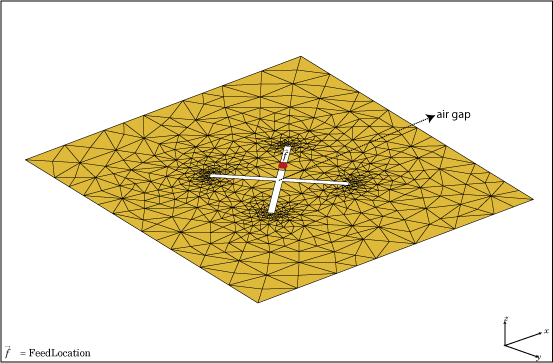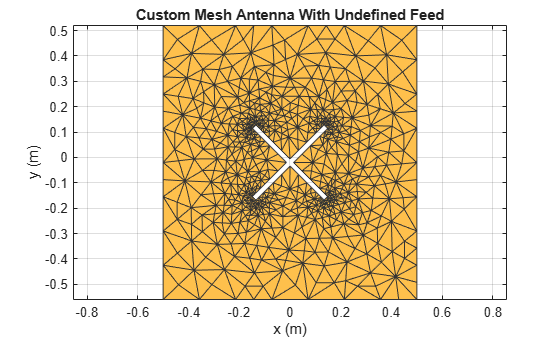# customAntennaMesh

Create 2-D custom mesh antenna on xy-plane

## Description

The `customAntennaMesh` object creates an antenna represented by a 2-D custom mesh on the xy-plane. You can provide an arbitrary antenna mesh to the Antenna Toolbox™ and analyze this mesh as a custom antenna for port and field characteristics.## Creation

### Description

example

````customantenna = customAntennaMesh(points,triangles)` creates a 2-D antenna represented by a custom mesh, based on the specified points and triangles.```

### Input Arguments

expand all

Points in a custom mesh, specified as a `2-by-N` or `3-by-N` integer matrix of Cartesian coordinates in meters. N is the number of points. In case you specify a `3xN` integer matrix, the Z-coordinate must be zero or a constant value. This value sets the `'Points'` property in the custom antenna mesh.

Example: `[0 1 0 1;0 1 1 0]`

Data Types: `double`

Triangles in the mesh, specified as a `4-by-M` integer matrix. M is the number of triangles. The first three rows are indices to the points matrix and represent the vertices of each triangle. The fourth row is a domain number useful for identifying separate parts of an antenna. This value sets the `'Triangles'` property in the custom antenna mesh.

Data Types: `double`

## Properties

expand all

Points in a custom mesh, specified as a `2-by-N` or `3-by-N` integer matrix of Cartesian coordinates in meters. N is the number of points.

Example: `[0.1 0.2 0]`

Data Types: `double`

Triangles in the mesh, specified as a `4-by-M` integer matrix. M is the number of triangles.

Data Types: `double`

Type of the metal used as a conductor, specified in the metal material object. You can choose any metal from the `MetalCatalog` or specify a metal of your choice. For more information, see `metal`. For more information on metal conductor meshing, see Meshing.

Example: `metal("Copper)`

Tilt angle of the antenna in degrees, specified as a scalar or vector. For more information, see Rotate Antennas and Arrays.

Example: `90`

Example: `Tilt=[90 90]`,`TiltAxis=[0 1 0;0 1 1]` tilts the antenna at 90 degrees about the two axes defined by the vectors.

Data Types: `double`

Tilt axis of the antenna, specified as one of these values:

• Three-element vector of Cartesian coordinates in meters. In this case, each coordinate in the vector starts at the origin and lies along the specified points on the x-, y-, and z-axes.

• Two points in space, specified as a 2-by-3 matrix corresponding to two three-element vectors of Cartesian coordinates. In this case, the antenna rotates around the line joining the two points.

• `"x"`, `"y"`, or `"z"` to describe a rotation about the x-, y-, or z-axis, respectively.

Example: `[0 1 0]`

Example: `[0 0 0;0 1 0]`

Example: `"Z"`

Data Types: `double` | `string`

## Object Functions

 `show` Display antenna, array structures, shapes, or platform `info` Display information about antenna or array `axialRatio` Axial ratio of antenna `beamwidth` Beamwidth of antenna `charge` Charge distribution on antenna or array surface `createFeed` Create feed location for custom antenna `current` Current distribution on antenna or array surface `efficiency` Radiation efficiency of antenna `EHfields` Electric and magnetic fields of antennas or embedded electric and magnetic fields of antenna element in arrays `impedance` Input impedance of antenna or scan impedance of array `mesh` Mesh properties of metal, dielectric antenna, or array structure `meshconfig` Change meshing mode of antenna, array, custom antenna, custom array, or custom geometry `pattern` Plot radiation pattern and phase of antenna or array or embedded pattern of antenna element in array `patternAzimuth` Azimuth plane radiation pattern of antenna or array `patternElevation` Elevation plane radiation pattern of antenna or array `returnLoss` Return loss of antenna or scan return loss of array `sparameters` Calculate S-parameters for antennas and antenna arrays `vswr` Voltage standing wave ratio (VSWR) of antenna or array element

## Examples

collapse all

Load a custom planar mesh. Create the antenna and antenna feed. View the custom planar mesh antenna and calculate the impedance at 100 MHz.

```load planarmesh.mat; c = customAntennaMesh(p,t); show(c)``````createFeed(c,[0.07,0.01],[0.05,0.05]); Z = impedance(c,100e6)```
```Z = 0.5091 +57.2102i ```

 Balanis, C.A. Antenna Theory: Analysis and Design. 3rd Ed. New York: Wiley, 2005.